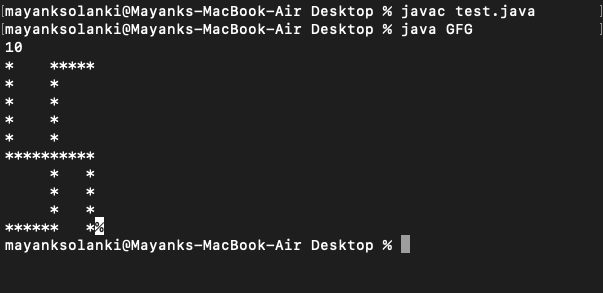# Java Program to Print Swastika Sign By Taking User Input

• Difficulty Level : Easy
• Last Updated : 31 Aug, 2021

Take as input N, an odd number (>=5). Print the following pattern as given below for N = 7. Here the input format is the value of N and output results out in swastika pattern printing.

Illustration:

Attention reader! Don’t stop learning now. Get hold of all the important Java Foundation and Collections concepts with the Fundamentals of Java and Java Collections Course at a student-friendly price and become industry ready. To complete your preparation from learning a language to DS Algo and many more,  please refer Complete Interview Preparation Course.

```Input Format: Enter value of N ( >=5 )
Constraints: 5 <= N <= 99```
`Output Format: Print the required pattern.`
```*     * * * *

*     *

*     *

* * * * * * *

*     *

*     *

* * * *       *```

Let us thinks of a way out in order to figure out by listing the logic prior to writing any code in order to generate the desired pattern.

Procedure:

1. First, take an input (N) from the user.
2. Initialize rows and cols as 1 to make loops in for the statement.
3. In the first row, we definitely know we have to print a * then give some spaces till N/2, and then again print stars till N.
4. In the second to N/2 rows, first, we need to print a * then spaces till N/2 (that it , we don’t need to provide spaces here)
5. In the (N/2)+1 th row, just print * till N.
6. Again in (N/2) +2th row to N -1, we need to first print spaces till N/2 and then print a * and the spaces till N-1 and finally a * at the end
7. In the final row, we need to print * till (N/2) + 1 and then print spaces till N-1 now and then finally ending it a *.
8. Lastly do not forget to add System.out.println() to add a blank line after each line of work

Now let us implement the some by writing the core for the same.

Example

## Java

 `// Java program to Illustrate Swastika Sign Pattern Printing ` ` `  `// Main class ` `// SwastikaSign ` `class` `GFG { ` ` `  `    ``// Main driver method ` `    ``public` `static` `void` `main(String args[]) ` `    ``{ ` ` `  `        ``// Creating an object of Scanner clas to read input ` `        ``// from user ` `        ``Scanner sc = ``new` `Scanner(System.in); ` ` `  `        ``// Reading number N from user ` `        ``int` `N = sc.nextInt(); ` `        ``// Custom setting the rows to be 10 to ` `        ``// understand the output ` ` `  `        ``// Initializing rows to unity initially ` `        ``int` `rows = ``1``; ` ` `  `        ``// This condition holds true ` `        ``// Till rows are lesser than input number from user ` `        ``while` `(rows <= N) { ` ` `  `            ``if` `(rows == ``1``) { ` ` `  `                ``// Start work ` `                ``System.out.print(``"*"``); ` ` `  `                ``// Space work ` `                ``for` `(``int` `csp = ``1``; csp < N / ``2``; csp++) ` `                    ``System.out.print(``" "``); ` ` `  `                ``// Star work ` `                ``for` `(``int` `cst = (N / ``2``) + ``1``; cst <= N; cst++) ` ` `  `                    ``System.out.print(``"*"``); ` `                ``System.out.println(); ` `            ``} ` ` `  `            ``else` `if` `(rows <= N / ``2` `&& rows > ``1``) { ` `                ``// Star work ` `                ``System.out.print(``"*"``); ` ` `  `                ``// Space work ` `                ``for` `(``int` `csp = ``1``; csp < N / ``2``; csp++) ` ` `  `                    ``System.out.print(``" "``); ` `                ``System.out.print(``"*"``); ` `                ``System.out.println(); ` `            ``} ` ` `  `            ``else` `if` `(rows == (N / ``2``) + ``1``) { ` ` `  `                ``for` `(``int` `cstt = ``1``; cstt <= N; cstt++) ` ` `  `                    ``System.out.print(``"*"``); ` `                ``System.out.println(); ` `            ``} ` ` `  `            ``else` `if` `(rows <= N - ``1` `&& rows > (N / ``2``) + ``1``) { ` ` `  `                ``// Space work ` `                ``for` `(``int` `csp = ``1``; csp <= N / ``2``; csp++) ` `                    ``System.out.print(``" "``); ` ` `  `                ``// Star work ` `                ``System.out.print(``"*"``); ` ` `  `                ``// Space work ` `                ``for` `(``int` `cspp = ``1` `+ (N / ``2``); cspp < N - ``1``; ` `                     ``cspp++) ` ` `  `                    ``System.out.print(``" "``); ` ` `  `                ``// Star work ` `                ``System.out.print(``"*"``); ` `                ``System.out.println(); ` `            ``} ` `            ``else` `{ ` ` `  `                ``for` `(``int` `csttt = ``1``; csttt <= (N / ``2``) + ``1``; ` `                     ``csttt++) ` ` `  `                    ``System.out.print(``"*"``); ` ` `  `                ``for` `(``int` `csppp = (N / ``2``) + ``2``; ` `                     ``csppp <= N - ``1``; csppp++) ` ` `  `                    ``System.out.print(``" "``); ` `                ``System.out.print(``"*"``); ` `            ``} ` `            ``rows++; ` `        ``} ` `    ``} ` `}`

Output:Note: One can also use BufferedReader class if you want to take input from user to enter the number of rows here.

My Personal Notes arrow_drop_up
Recommended Articles
Page :